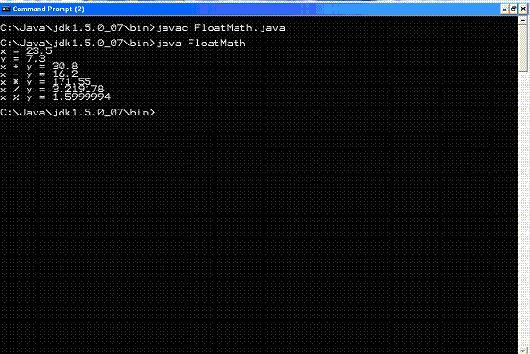## Core Java

Java Evolution
Overview of Java Language
Constant, Variable and Datatype
Operator and Expression
Decision making, Branching and Looping
Class Object and Method
Array and String
Inheritance
Package
Exception Handling
Applet
Managing Input/Output Files in Java

# Operator and Expression

### Floating point Operators

 There are three types of operations that can be performed on floating-point numbers: 1. unary, 2. binary, 3. and relational Unary operators act only on single floating-point numbers, binary operators act on pairs of floating-point numbers. Both unary and binary floating-point operators return floating-point results. Relational operators, act on two floating-point numbers but return a boolean result. Unary and binary floating-point operators return a float type if both operands are of type float. If one or both of the operands are of type double, however, the result of the operation is of type double.

Unary Floating-Point Operators
The unary floating point operators act on a single floating-point number. Lists of the unary floating-point operators.

The unary floating-point operators.
 Description Operator Increment ++ Decrement --

The only two unary floating-point operators are the increment and decrement operators. These two operators respectively add and subtract 1.0 from their floating-point operand.

Binary Floating-Point Operators
The binary floating-point operators act on a pair of floating-point numbers. Lists of the binary floating-point operators.

The binary floating-point operators.
 Description Operator Addition + Subtraction - Multiplication * Division / Modulus %

The binary floating-point operators consist of the four traditional binary operations (+, -, *, /), along with the modulus operator (%).
 The FloatMath class. class FloatMath { public static void main (String args[]) { float x = 23.5F, y = 7.3F; System.out.println("x = " + x); System.out.println("y = " + y); System.out.println("x + y = " + (x + y)); System.out.println("x - y = " + (x - y)); System.out.println("x * y = " + (x * y)); System.out.println("x / y = " + (x / y)); System.out.println("x % y = " + (x % y)); } } The output of FloatMath follows: x = 23.5 y = 7.3 x + y = 30.8 x - y = 16.2 x * y = 171.55 x / y = 3.21918 x % y = 1.6 Output: